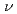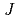Electric field of laser pulses which gives maximum selectivity in the isotope-selective rovibrational excitation of lithium chloride molecules is calculated. Applying the optimal control theory, we calculate optimal electric field to produce mixture of LiCl-35 (=0,=0) and LiCl-37 (=1,=1) from molecular ensemble of LiCl-35 (=0,=0) and LiCl-37 (=0,=0). As a result, it is found that electric field which permit rotational excitations only gives high yield in the selective excitation compared to the electric field which permit both rotational and vibrational excitations.